# GSEB Class 11 Statistics Notes Chapter 4 Measures of Dispersion

This GSEB Class 11 Commerce Statistics Notes Chapter 4 Measures of Dispersion covers all the important topics and concepts as mentioned in the chapter.

## Measures of Dispersion Class 11 GSEB Notes

Meaning of Dispersion:
Dispersion:

• A measure which shows how far the observations of the data are scattered from the measure of average is called dispersion.
• It is a precise measure of variation of population observations.
• According to Spiegel, “The degree to which numerical data tend to spread about an average value is called variation or dispersion of the data.”

Characteristics of Dispersion: Some desirable characteristics of dispersion are as follows:

• Its definition is clear and precise,
• It is simple to understand and easy to calculate,
• Based on all the observations of the data,
• Less affected by extreme values
• Suitable for algebric operations
• Less affected by sampling and

Types of Dispersion:

• Absolute Dispersion: A measure of dispersion which is expressed in the same units of observation of the data. If the units of observations of the data is ₹ than absolute dispersion is expressed in ₹. It cannot be used for comparing dispersion of observations of two or more data.
• Relative Dispersion: A measure of dispersion which is free from the unit of measurement is called relative measure of dispersion. It can be used for comparing the dispersion of observations of the two or more data having different units of measurement. Relative measure of dispersion is known as coefficient of dispersion. It is ratio of absolute dispersion to the average measure of the data.

Measures of Dispersion:

• Range: The difference between the highest and the lowest observation of the data is called Range and it is denoted by R.
• Quartile Deviation: Quartile deviation is the value obtained by dividing the difference between the third quartile Q3 and the first quartile Q1 by 2. It is denoted by Qd. It is also known as the semi-inter-quartile range.
• Mean Deviation: The mean of the absolute deviations of the observations of a data from its mean is called mean deviation. It is denoted by MD.
• Standard Deviation: The positive square root of the mean of the squares of deviations of the observations of a data measured from the mean. It is denoted by ‘s’.

Variance:
The mean of the squares of deviations of observation of a data measured from the mean. It is denoted by s2.Relative Measures of Dispersion:
1. Coefficient of Range:
The value obtained by dividing the measure of range by sum of the highest value and the lowest value is called the coefficient of range.

Coefficient of range = $$\frac{\text { highest value }-\text { lowest value }}{\text { highest value }+\text { lowest value }}=\frac{x_{\mathrm{H}}-x_{\mathrm{L}}}{x_{\mathrm{H}}+x_{\mathrm{L}}}$$

2. Coefficient of Quartile Deviation:
The value obtained by dividing the measure ‘ of quartifes deviation by the average of third quartile and first quartile is called the coefficient of quartile deviation.

Coefficient of quartile deviation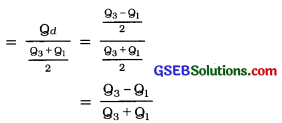3. Coefficient of Mean Deviation:
The value obtained by dividing the mean deviation by mean is called the coefficient of mean deviation.
Coefficient of mean deviation = $$\frac{\text { mean deviation }}{\text { mean }}=\frac{\mathrm{MD}}{\bar{x}}$$

4. Coefficient of Standard Deviation: The value obtained by dividing the standard deviation by mean is called the coefficient of standard deviation.
Coefficient of standard deviation = $$\frac{\text { standard deviation }}{\text { mean }}=\frac{s}{\bar{x}}$$

5. Coefficient of Variation: The value obtained by multiplying the coefficient of standard deviation by 100 is called the coefficient of variation. It is a percentage relative measure of standard deviation.
Coefficient of variation = coefficient of standard deviation × 100
= $$\frac{s}{\bar{x}}$$ × 100

• It is very much useful for comparing the dispersion of two or more data.
• Lesser the coefficient of variation higher the stability and consistency of the data and the dispersion is less.
• Higher the coefficient of variation lesser the stability and consistency of the data and the dispersion is more.

Combined Standard Deviation:
If mean, standard deviation and number of observations of two groups are given, then the standard deviation obtained for combined group of the given two groups is called combined standard deviation. It is denoted by sc.

Important Formulae
1. Range:

 Ungrouped data Grouped data Discrete frequency distribution Continuous frequency distribution R = xH – xL Where xH = Highest value xL = Lowest value R = xH – xL Where xH = Highest value xL = Lowest value R = xH – xL Where xH = Highest value xL = Lowest value Coefficient of range = $$\frac{x_{\mathrm{H}}-x_{\mathrm{L}}}{x_{\mathrm{H}}+x_{\mathrm{L}}}=\frac{\mathrm{R}}{x_{\mathrm{H}}+x_{\mathrm{L}}}$$

2. Quartile deviation
Ungrouped and grouped data:
Qd = $$\frac{\mathrm{Q}_{3}-\mathrm{Q}_{1}}{2}$$
Coefficient of quartile deviation = $$\frac{\mathrm{Q}_{3}-\mathrm{Q}_{1}}{\mathrm{Q}_{3}+\mathrm{Q}_{1}}$$
Where, Q3 = Third quartile;
Q1 = First quartile;
Qd = Quartile deviation

3. Mean deviation
Ungrouped data:
MD = $$\frac{\Sigma|x-\bar{x}|}{n}$$
Where, |x – x̄| = Absolute difference between observations and mean
n = Total number of observations

Grouped data:

 Discrete frequency distribution: Continuous frequency distribution: MD = $$\frac{\sum f|x-\bar{x}|}{n}$$ Where, x̄ = Mean x = Observation f = Frequency of observation n = Σf = Sum of frequencies MD = $$\frac{\sum f|x-\bar{x}|}{n}$$ Where, x̄ = Mean x = Mid value of class f = Frequency of observation n = Σf = Sum of frequencies Coefficient of Mean variation = $$\frac{\mathrm{MD}}{\bar{x}}$$4. Standard deviation:
Ungrouped data: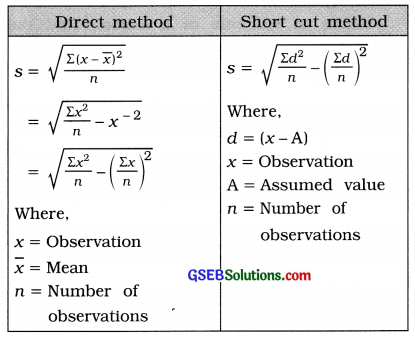Grouped data: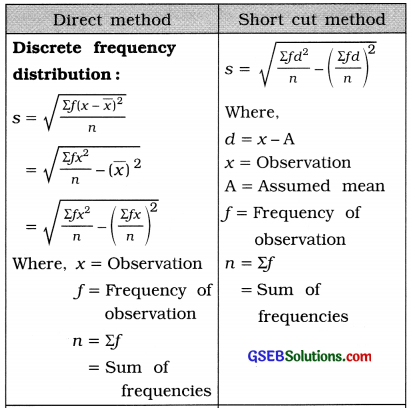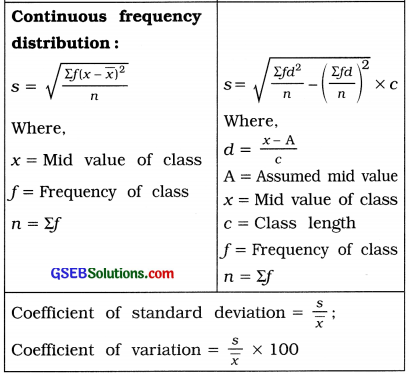5. Variance: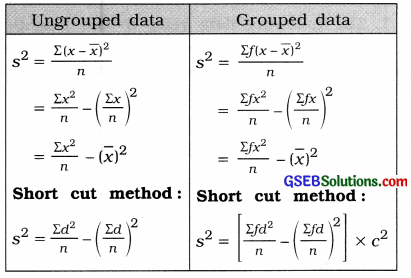6. Combined standard deviation
sc = $$\sqrt{\frac{n_{1}\left(s_{1}^{2}+d_{1}^{2}\right)+n_{2}\left(s_{2}^{2}+d_{2}^{2}\right)}{n_{1}+n_{2}}}$$;
c = $$\frac{n_{1} \bar{x}_{1}+n_{2} \bar{x}_{2}}{n_{1}+n_{2}}$$
Where,
n1 = Number of observations of first group
s1 = S.d. of first group
d1 = x̄1 – x̄c
1 = Mean of first group
c = Combined mean of both the groups
n2 = Number of observations of second group
s2 = S.d. of second group
d2 = x̄2 – x̄c
2 = Mean of second group

7. Relative measures of dispersion
Range:
Coefficient of range = $$\frac{x_{\mathrm{H}}-x_{\mathrm{L}}}{x_{\mathrm{H}}+x_{\mathrm{L}}}=\frac{\mathrm{R}}{x_{\mathrm{H}}+x_{\mathrm{L}}}$$

• Quartile deviation:
Coefficient of quartile deviation = $$\frac{Q_{3}-Q_{1}}{Q_{3}+Q_{1}}$$
• Mean deviation:
Coefficient of mean deviation = $$\frac{\text { MD }}{\bar{x}}$$
• Standard deviation:
Coefficient of variation = $$\frac{s}{\bar{x}}$$ × 1oo